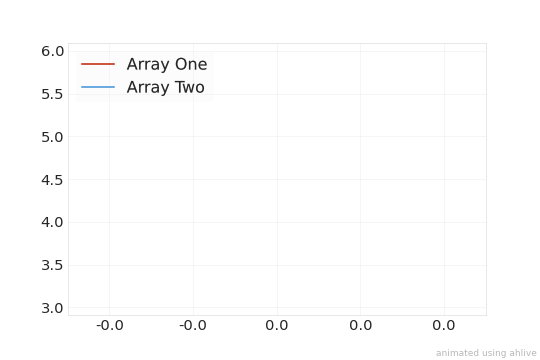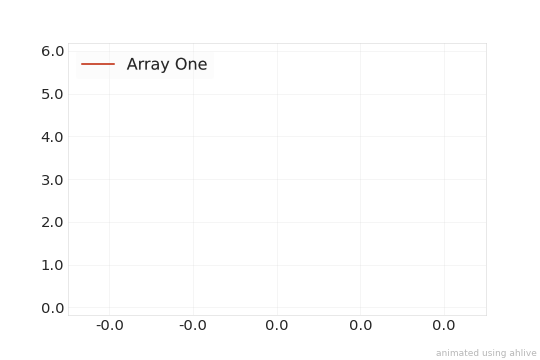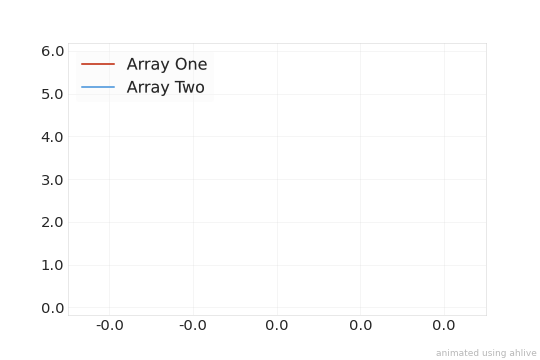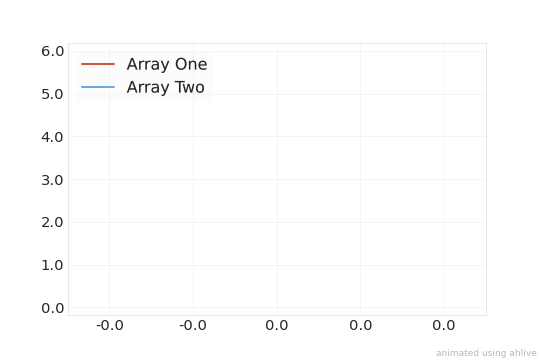Merging¶

ahlive allows data to be combined into a single figure to let the animations run simultaneously.

merging functions¶

ahlive has these functions for merging data:

ahlive function

method

operator

description

ah.overlay

overlay

*

stack plots over one another into a single subplot

-

like overlay, but animates the data serially, one after another

ah.stagger

stagger

**

like overlay, but animates the data in a staggered fashion, step by step

ah.slide

slide

//

like overlay, but delays the latter datasets by a single frame

ah.layout

layout

+ /

arrange plots horizontally or vertically as multiple subplots

ah.merge

n/a

n/a

a generic method for all merges by specifying join

overlay¶

To overlay, simply multiply the objects.

This results in an ah.Data object that contains multiple items within the wrapped xr.Dataset.

:
import ahlive as ah
arr1 = ah.Array([0, 1, 2], [4, 5, 4], label='Array One', chart='line')
arr2 = ah.Array([0, 1, 2], [3, 5, 6], label='Array Two', chart='line')
# equivalently arr1.overlay(arr2) or ah.overlay([arr1, arr2])
overlay = arr1 * arr2
print(overlay)
overlay.render()
<ahlive.Data>
Subplot:         (1, 1)
Dimensions:      (item: 2, state: 3)
Data variables:
x        (item, state) float64 0.0 1.0 2.0 0.0 1.0 2.0
y        (item, state) float64 4.0 5.0 4.0 3.0 5.0 6.0
chart    (item) <U4 'line' 'line'
label    (item, state) object 'Array One' 'Array One' ... 'Array Two'
group    (item) <U1 '' ''
Attributes (0/49):

:To cascade, simply subtract the objects.

Similar to overlay, this results in an ah.Data object that contains multiple items within the wrapped xr.Dataset, but the difference is that the first ah.Data object’s animation concludes before the second ah.Data object is starts.

:
import ahlive as ah
arr1 = ah.Array([0, 1, 2], [0, 5, 4], label='Array One', chart='line')
arr2 = ah.Array([0, 1, 2], [3, 5, 6], label='Array Two', chart='line')
<ahlive.Data>
Subplot:         (1, 1)
Dimensions:      (item: 2, state: 6)
Data variables:
x        (item, state) float64 0.0 1.0 2.0 2.0 2.0 ... nan nan 0.0 1.0 2.0
y        (item, state) float64 0.0 5.0 4.0 4.0 4.0 ... nan nan 3.0 5.0 6.0
chart    (item) object 'line' 'line'
label    (item, state) object 'Array One' 'Array One' ... 'Array Two'
group    (item) object '' ''
Attributes (0/49):

:stagger¶

To stagger, use the exponent symbol on the objects.

Similar to overlay and cascade, this results in an ah.Data object that contains additional items and states within the wrapped xr.Dataset, but the difference is that the first ah.Data and the second ah.Data objects take turns animating.

:
import ahlive as ah
arr1 = ah.Array([0, 1, 2], [0, 5, 4], label='Array One', chart='line')
arr2 = ah.Array([0, 1, 2], [3, 5, 6], label='Array Two', chart='line')
# equivalently arr1.stagger(arr2) or ah.stagger([arr1, arr2])
stagger = arr1 ** arr2
print(stagger)
stagger.render()
<ahlive.Data>
Subplot:         (1, 1)
Dimensions:      (item: 2, state: 6)
Data variables:
x        (item, state) float64 0.0 1.0 1.0 2.0 2.0 ... 0.0 1.0 1.0 2.0 2.0
y        (item, state) float64 0.0 5.0 5.0 4.0 4.0 ... 3.0 5.0 5.0 6.0 6.0
chart    (item) object 'line' 'line'
label    (item, state) object 'Array One' 'Array One' ... 'Array Two'
group    (item) object '' ''
Attributes (0/49):

:slide¶

To slide, use the floor division symbol on the objects.

Similar to overlay, cascade, and stagger, this results in an ah.Data object that contains additional items and states within the wrapped xr.Dataset, but the latter ah.Data objects’ frames are offset by one and animated simultaneously.

:
import ahlive as ah
arr1 = ah.Array([0, 1, 2], [0, 5, 4], label='Array One', chart='line')
arr2 = ah.Array([0, 1, 2], [3, 5, 6], label='Array Two', chart='line')
# equivalently arr1.slide(arr2) or ah.slide([arr1, arr2])
slide = arr1 // arr2
print(slide)
slide.render()
<ahlive.Data>
Subplot:         (1, 1)
Dimensions:      (item: 2, state: 4)
Data variables:
x        (item, state) float64 0.0 1.0 2.0 2.0 0.0 0.0 1.0 2.0
y        (item, state) float64 0.0 5.0 4.0 4.0 3.0 3.0 5.0 6.0
chart    (item) object 'line' 'line'
label    (item, state) object 'Array One' 'Array One' ... 'Array Two'
group    (item) object '' ''
Attributes (0/49):

:layout¶

To layout, simply add the objects to lay them out horizontally or divide the objects to lay them out vertically.

This results in an ah.Data object that contains multiple keys within the wrapped dict.

:
import ahlive as ah
arr1 = ah.Array([0, 1], [4, 5], title='Array One', chart='line')
arr2 = ah.Array([0, 1], [3, 5], title='Array Two', chart='line')
arr3 = ah.Array([0, 1], [7, 8], title='Array Three', chart='line')
layout = (arr1 + arr2) / arr3
print(layout)
layout.render()
<ahlive.Data>
Subplot:         (1, 1)
Dimensions:      (item: 1, state: 2)
Data variables:
x        (item, state) float64 0.0 1.0
y        (item, state) float64 4.0 5.0
chart    (item) <U4 'line'
label    (item, state) <U1 '' ''
group    (item) <U1 ''
Attributes (1/49):
title_kwds   {'label': 'Array One'}

Subplot:         (1, 2)
Dimensions:      (item: 1, state: 2)
Data variables:
x        (item, state) float64 0.0 1.0
y        (item, state) float64 3.0 5.0
chart    (item) <U4 'line'
label    (item, state) <U1 '' ''
group    (item) <U1 ''
Attributes (1/49):
title_kwds   {'label': 'Array Two'}

Subplot:         (2, 1)
Dimensions:      (item: 1, state: 2)
Data variables:
x        (item, state) float64 0.0 1.0
y        (item, state) float64 7.0 8.0
chart    (item) <U4 'line'
label    (item, state) <U1 '' ''
group    (item) <U1 ''
Attributes (1/49):
title_kwds   {'label': 'Array Three'}

: